# 7 Segment Display Interfacing with Raspberry Pi

Published  June 18, 2016   1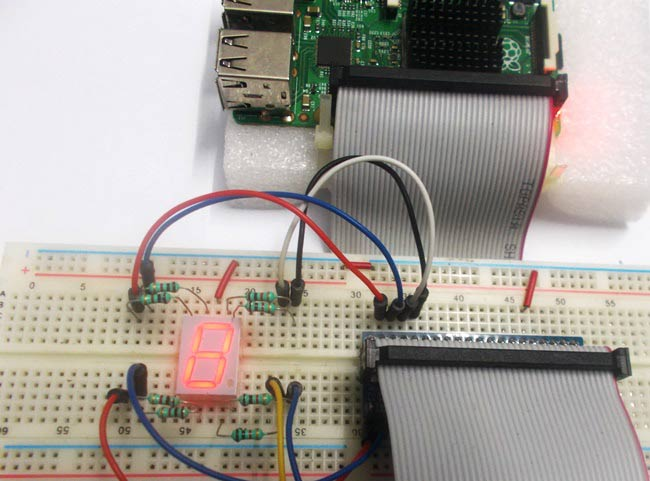Raspberry Pi is an ARM architecture processor based board designed for electronic engineers and hobbyists. The PI is one of most trusted project development platforms out there now. With higher processor speed and 1 GB RAM, the PI can be used for many high profile projects like Image processing and IoT.

For doing any of high profile projects, one need to understand the basic functions of PI. We will be covering all the basic functionalities of Raspberry Pi in these tutorials. In each tutorial we will discuss one of functions of PI. By the end of this Raspberry Pi Tutorials Series, you will be able to learn Raspberry Pi and make good projects by yourself. Go through below tutorials:

In this tutorial, we are going to do Raspberry Pi 7 segment display interfacing. Seven Segment displays are the cheapest for a display unit. A couple of these segments stacked together could be used to display temperature, counter value etc. We will connect the 7 segment display unit to GPIO of PI and control them to display digits accordingly. After that we will write a program in PYTHON for seven segment display to counts from 0-9 and resets itself to zero.

### Seven Segment Display:

There are different types and sizes of 7 Segment Displays. We have covered Seven Segment working in detail here. Basically there are two types of 7 Segment, Common Anode type (Common Positive or Common VCC) and Common Cathode type (Common Negative or Common Ground).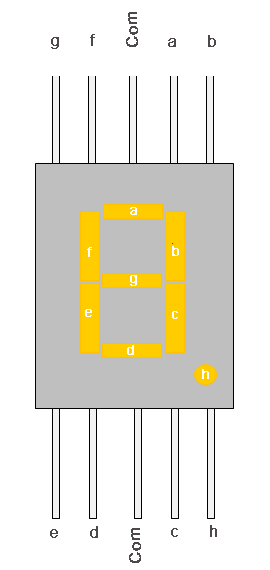Common Anode (CA): In this all the Negative terminals (cathode) of all the 8 LEDs are connected together (see diagram below), named as COM. And all the positive terminals are left alone.

Common Cathode (CC): In this all the positive terminals (Anodes) of all the 8 LEDs are connected together, named as COM. And all the negative thermals are left alone.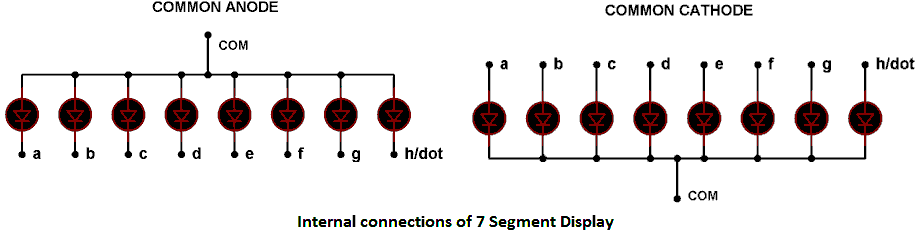These CC and CA seven segment displays come in very handy while multiplexing several cells together. In our tutorial we will use CC or Common Cathode Seven Segment Display.

We have already Interfaced 7 segment with 8051, with Arduino and with AVR. We have also used 7 segment display in many of our Projects.

We will discuss a bit about Raspberry Pi GPIO before going any further,

There are 40 GPIO output pins in Raspberry Pi 2. But out of 40, only 26 GPIO pins (GPIO2 to GPIO27) can be programmed, see the figure below. Some of these pins perform some special functions. With special GPIO put aside, we have 17 GPIO remaining.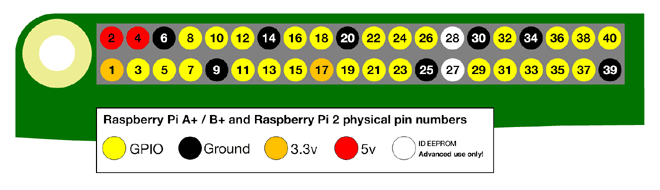The GPIO’s (pin 1 or 17) +3.3V signal is enough to drive the 7 Segment Display. To provide current limit, we will use 1KΩ resistor for each segment as shown in the Circuit Diagram.

To know more about GPIO pins and their current outputs, go through: LED Blinking with Raspberry Pi

### Components Required:

Here we are using Raspberry Pi 2 Model B with Raspbian Jessie OS. All the basic Hardware and Software requirements are previously discussed, you can look it up in the Raspberry Pi Introduction, other than that we need:

• Connecting pins
• Common Cathode 7 segment display (LT543)
• 1KΩresistor (8 pieces)

### Circuit and Working Explanation: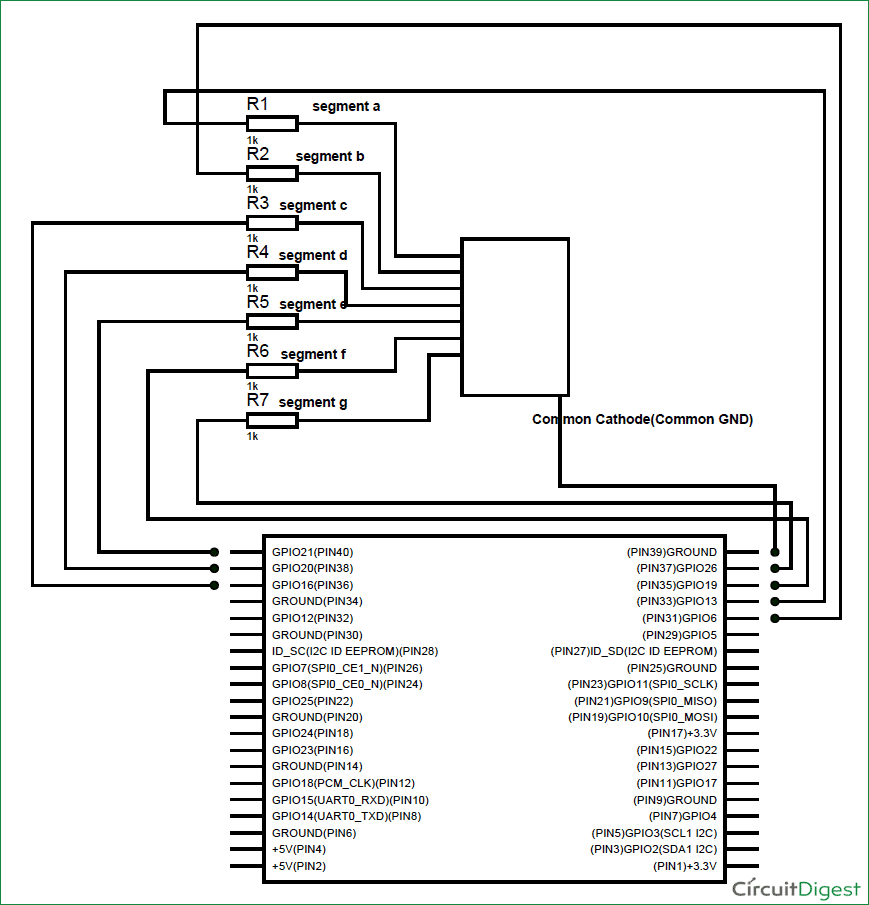The connections, which are done for Interfacing 7 segment display to Raspberry Pi, are given below. We have used Common Cathode 7 Segment here:

PIN1 or e ------------------ GPIO21

PIN2 or d ------------------ GPIO20

PIN4 or c ------------------ GPIO16

PIN5 or h or DP ---------- GPIO 12 //not compulsory as we are not using decimal point

PIN6 or b ------------------GPIO6

PIN7 or a ------------------GPIO13

PIN9 or f ------------------ GPIO19

PIN10 or g ---------------- GPIO26

PIN3 or PIN8 -------------connected to Ground

So we will be using 8 GPIO pins of PI as an 8bit PORT. In here GPIO13 is being LSB (Least Significant Bit) and GPIO 12 being MSB (Most Significant Bit).

Now, if we want to display Number “1”, we need to power segments B and C. To power segment B and C, we need to power GPIO6 and GPIO16. So the byte for ‘PORT’ function will be 0b00000110 and the hex value of ‘PORT’ will be 0x06. With both the pins high we get “1” on display.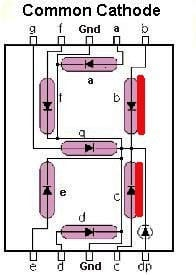We have written the values for each digit to be displayed and stored those values in a String of Characters named ‘DISPLAY’ (Check the Code section below). Then we have called those values one by one to show the corresponding digit on the display, using the Function ‘PORT’.

### Programming Explanation:

Once everything is connected as per the circuit diagram, we can turn ON the PI to write the program in PYHTON.

We will talk about few commands which we are going to use in PYHTON program,

We are going to import GPIO file from library, below function enables us to program GPIO pins of PI. We are also renaming “GPIO” to “IO”, so in the program whenever we want to refer to GPIO pins we will use the word ‘IO’.

`import RPi.GPIO as IO`

Sometimes, when the GPIO pins, which we are trying to use, might be doing some other functions. In that case, we will receive warnings while executing the program. Below command tells the PI to ignore the warnings and proceed with the program.

`IO.setwarnings(False)`

We can refer the GPIO pins of PI, either by pin number on board or by their function number. Like ‘PIN 29’ on the board is ‘GPIO5’. So we tell here either we are going to represent the pin here by ‘29’ or ‘5’.

`IO.setmode (IO.BCM)`

We are setting 8 GPIO pins as output pins, for Data and Control pins of LCD.

```IO.setup(13,IO.OUT)
IO.setup(6,IO.OUT)
IO.setup(16,IO.OUT)
IO.setup(20,IO.OUT)
IO.setup(21,IO.OUT)
IO.setup(19,IO.OUT)
IO.setup(26,IO.OUT)
IO.setup(12,IO.OUT)```

In case the condition in the braces is true, the statements inside the loop will be executed once. So if the bit0 of 8bit 'pin' is true, PIN13 will be HIGH, otherwise PIN13 will be LOW. We have eight ‘if else’ conditions for bit0 to bit7, so that appropriate LED, inside the 7 segment display, can be made High or Low, to display the corresponding Number.

```if(pin&0x01 == 0x01):
IO.output(13,1)
else:
IO.output(13,0)```

This command executes the loop 10 times, x being incremented from 0 to 9.

`for x in range(10):`

Below command is used as forever loop, with this command the statements inside this loop will be executed continuously.

`While 1:`

All the other functions and commands have been explained in below ‘Code’ section with the help of ‘Comments’.

After writing the program and executing it, the Raspberry Pi triggers the corresponding GPIOs to show the digit on 7 Segment Display. The program is written for the display to count from 0-9 continuously.

Code

import RPi.GPIO as IO            # calling for header file which helps us use GPIO’s of PI
import time                              # calling for time to provide delays in program

DISPLAY = [0x3F,0x06,0x5B,0x4F,0x66,0x6D,0x7D,0x07,0x7F,0x67]            # string of characters storing PORT values for each digit.

IO.setwarnings(False)            # do not show any warnings
IO.setmode (IO.BCM)           # programming the GPIO by BCM pin numbers. (like PIN29 as‘GPIO5’)

IO.setup(13,IO.OUT)             # initialize GPIO Pins as outputs
IO.setup(6,IO.OUT)
IO.setup(16,IO.OUT)
IO.setup(20,IO.OUT)
IO.setup(21,IO.OUT)
IO.setup(19,IO.OUT)
IO.setup(26,IO.OUT)
IO.setup(12,IO.OUT)

def PORT(pin):                    # assigning GPIO logic by taking 'pin' value
if(pin&0x01 == 0x01):
IO.output(13,1)            # if  bit0 of 8bit 'pin' is true, pull PIN13 high
else:
IO.output(13,0)            # if  bit0 of 8bit 'pin' is false, pull PIN13 low
if(pin&0x02 == 0x02):
IO.output(6,1)             # if  bit1 of 8bit 'pin' is true, pull PIN6 high
else:
IO.output(6,0)            #if  bit1 of 8bit 'pin' is false, pull PIN6 low
if(pin&0x04 == 0x04):
IO.output(16,1)
else:
IO.output(16,0)
if(pin&0x08 == 0x08):
IO.output(20,1)
else:
IO.output(20,0)
if(pin&0x10 == 0x10):
IO.output(21,1)
else:
IO.output(21,0)
if(pin&0x20 == 0x20):
IO.output(19,1)
else:
IO.output(19,0)
if(pin&0x40 == 0x40):
IO.output(26,1)
else:
IO.output(26,0)
if(pin&0x80 == 0x80):
IO.output(12,1)            # if  bit7 of 8bit 'pin' is true, pull PIN12 high
else:
IO.output(12,0)            # if  bit7 of 8bit 'pin' is false, pull PIN12 low

while 1:
for x in range(10):            # execute the loop ten times incrementing x value from zero to nine
pin = DISPLAY[x]        # assigning value to 'pin' for each digit
PORT(pin);                  # showing each digit on display
time.sleep(1)

Video

Tags